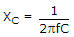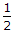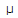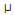# Electronics - Capacitors

## Why Electronics Capacitors?

In this section you can learn and practice Electronics Questions based on "Capacitors" and improve your skills in order to face the interview, competitive examination and various entrance test (CAT, GATE, GRE, MAT, Bank Exam, Railway Exam etc.) with full confidence.

## Where can I get Electronics Capacitors questions and answers with explanation?

IndiaBIX provides you lots of fully solved Electronics (Capacitors) questions and answers with Explanation. Solved examples with detailed answer description, explanation are given and it would be easy to understand. All students, freshers can download Electronics Capacitors quiz questions with answers as PDF files and eBooks.

## Where can I get Electronics Capacitors Interview Questions and Answers (objective type, multiple choice)?

Here you can find objective type Electronics Capacitors questions and answers for interview and entrance examination. Multiple choice and true or false type questions are also provided.

## How to solve Electronics Capacitors problems?

You can easily solve all kind of Electronics questions based on Capacitors by practicing the objective type exercises given below, also get shortcut methods to solve Electronics Capacitors problems.

### Exercise :: Capacitors - General Questions

1.

In a 20 Vac series RC circuit, if 20 V is measured across the resistor and 40 V is measured across the capacitor, the applied voltage is:

 A. 45 V B. 50 V C. 55 V D. 60 V

Explanation:

No answer description available for this question. Let us discuss.

2.

Select the equation below that represents the relationship between charge, capacitance, and voltage for a capacitor.

 A. Q = CV B. C = QV C.D. V = IR

Explanation:

No answer description available for this question. Let us discuss.

3.

When is a capacitor fully charged?

 A. when the voltage across its plates isof the voltage from ground to one of its plates B. when the current through the capacitor is the same as when the capacitor is discharged C. when the voltage across the plates is 0.707 of the input voltage D. when the current through the capacitor is directly proportional to the area of the plates

Explanation:

No answer description available for this question. Let us discuss.

4.What is the reactive power in the given circuit?

 A. 0 VAR B. 691VAR C. 44.23 mVAR D. 1.45 kVAR

Explanation:

No answer description available for this question. Let us discuss.

5.

What is the angle theta value for a 5.6F capacitor and a 50-ohm resistor in series with a 1.1 kHz, 5 Vac source?

 A. 27.3 degrees B. 62.7 degrees C. –27.3 degrees D. –62.7 degrees